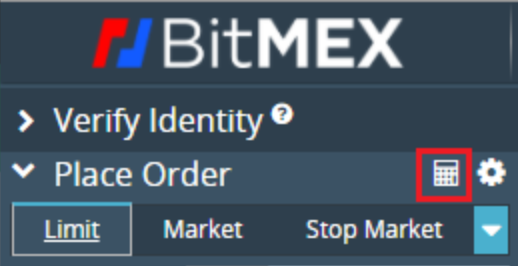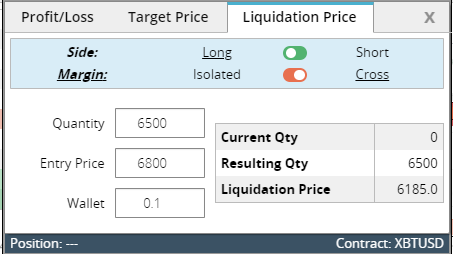The BitMEX calculator is located on the top left corner of the trading page, as seen below:The BitMEX calculator lets you calculate your profit/loss, liquidation price and ROE (Return on Equity)

The following is a breakdown of each section so you can calculate and understand each section of the calculator.

Profit/Loss

When it comes to calculating your profit or loss, it’s quite simple. From the image below, you can see how you can work out the amount of profit you can make trading on BitMEX.In this example, we have several inputs.

Quantity is the position size you are taking including the leverage you use. So if your equity is \$100 and you use 10x leverage, your position size will be \$1000.

Entry Price is the price at which you open the trade.

Exit Price is the target price at which you want to exit the trade.

Leverage is the multiplier of your initial investment that you use to increase your position up to 100x.

From this, you can see that a 10% price move, gives us 90.9% ROE and a profit of 0.0151 XBT.

However, if the price were to move in the other direction and go down 10%, your position would likely be taken over by the Liquidation Engine and your initial margin of 0.0169 would be lost.

ROE

Your ROE (Return on Equity) is how much you make compared to how much you put down.

For example, if you were to put down \$100 in equity and set the leverage at 10x, your position size would be \$1000 in total.

If the price of the underlying asset increases by 10%, then your position would be worth \$1100 and you would have made \$100 in profit.

Your ROE would then be 100%, as your equity is \$100 and your profit is also \$100.With the target price calculator above, you can calculate what target price is required to achieve the ROE you desire. So if you’re looking to make \$100 and you have \$100 of equity, entering inputs based on these assumptions will help you arrive at a target price of \$6666.5 with an average entry price of \$6000.

Liquidation Price

The term “liquidation” is probably the most popular term amongst traders on BitMEX. If you’ve traded on high leverage, you definitely want to avoid any messages containing the word and this can be done by always being fully aware of how the liquidation price of your position is calculated.

For those who fear liquidation, we recommend you reduce your leverage, which will in turn move your liquidation price further away from your entry price.The following is a breakdown of each section of the calculator above, so you can adjust it to your assumptions.

Quantity: 6500 - This is the number of contracts you plan to buy or sell.

Entry Price: \$6800 - The price of the asset at which you wish to open a position.

Wallet: 0.1 XBT - The amount of XBT in your wallet (applies when using Cross Margin)

With all of this information entered into the calculator, you can see you have a liquidation price on the bottom right of \$6185. So if the price of XBT reaches the price of \$6185, then the Liquidation Engine will take over your position and all XBT in your wallet will be lost.

To move your liquidation price further away, you can adjust the quantity to a lower amount, For instance, if you adjust your position size in half from 6500 to 3250, you would arrive at a new liquidation price of \$5648 and this saves you from ending up being liquidated.The difference between Isolated Margin and Cross Margin in the event of a liquidation

If you are using Isolated Margin, margin assigned to a position is restricted to the amount assigned to the position. For example, if you assign \$100 to a position in Isolated Margin, \$100 is the maximum amount you could lose if you were liquidated.

Cross Margin, also known as “Spread Margin”, is a margin method that utilises the full amount of funds in the Available Balance to avoid liquidations - Any realised PNL from other positions can also aid in providing margin to a losing position. Therefore, when using Cross Margin, all your funds in your Available Balance will be lost if your position is liquidated.